# The SURVEYFREQ Procedure

#### Odds Ratio and Relative Risks

The OR option provides estimates of the odds ratio, the column 1 relative risk, and the column 2 relative risk fortables, together with their confidence limits.

##### Odds Ratio

For atable, the odds of a positive (column 1) response in row 1 is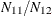. Similarly, the odds of a positive response in row 2 is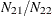. The odds ratio is formed as the ratio of the row 1 odds to the row 2 odds. The estimate of the odds ratio is computed as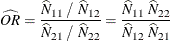The value of the odds ratio can be any nonnegative number. When the row and column variables are independent, the true value of the odds ratio equals 1. An odds ratio greater than 1 indicates that the odds of a positive response are higher in row 1 than in row 2. An odds ratio less than 1 indicates that the odds of positive response are higher in row 2. The strength of association increases with the deviation from 1. For details, see Stokes, Davis, and Koch (2000); Agresti (2007).

PROC SURVEYFREQ constructs confidence limits for the odds ratio by using the log transform. The% confidence limits for the odds ratio are computed as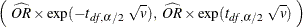where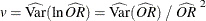is the estimate of the variance of the log odds ratio andis the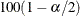percentile of the t distribution with df degrees of freedom. (For more information, see the section Degrees of Freedom.) The value of the confidence coefficientis determined by the ALPHA= option, which by default equals 0.05 and produces 95% confidence limits.

If you request BRR variance estimation (VARMETHOD=BRR), PROC SURVEYFREQ estimates the variance of the odds ratio as described in the section Balanced Repeated Replication (BRR). If you request jackknife variance estimation (VARMETHOD=JACKKNIFE), the procedure estimates the variance as described in the section The Jackknife Method.

If you do not specify the VARMETHOD= option or a REPWEIGHTS statement, the default variance estimation method is Taylor series (VARMETHOD=TAYLOR). By using Taylor series linearization, the variance estimate for the odds ratio can be expressed aswhere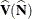is the covariance matrix of the estimates of the cell totals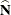,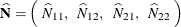andis an array that contains the partial derivatives of the odds ratio with respect to the elements of. The section Covariance of Totals describes the computation of. The arrayis computed as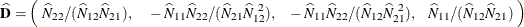##### Relative Risks

For atable, the column 1 relative risk is the ratio of the column 1 risks for row 1 to row 2. As described in the section Risks and Risk Difference, the column 1 risk for row 1 is the proportion of row 1 observations classified in column 1, and the column 1 risk for row 2 is the proportion of row 2 observations classified in column 1. The estimate of the column 1 relative risk is computed as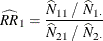Similarly, the estimate of the column 2 relative risk is computed asA relative risk greater than 1 indicates that the probability of positive response is greater in row 1 than in row 2. Similarly, a relative risk less than 1 indicates that the probability of positive response is less in row 1 than in row 2. The strength of association increases with the deviation from 1. For more information, see Stokes, Davis, and Koch (2000); Agresti (2007).

PROC SURVEYFREQ constructs confidence limits for the relative risk by using the log transform, which is similar to the odds ratio computations described previously. The% confidence limits for the column 1 relative risk are computed as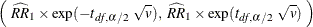whereis the estimate of the variance of the log column 1 relative risk andis thepercentile of the t distribution with df degrees of freedom. (For more information, see the section Degrees of Freedom.) The value of the confidence coefficientis determined by the ALPHA= option, which by default equals 0.05 and produces 95% confidence limits.

If you request BRR variance estimation (VARMETHOD=BRR), PROC SURVEYFREQ estimates the variance of the column 1 relative risk as described in the section Balanced Repeated Replication (BRR). If you request jackknife variance estimation (VARMETHOD=JACKKNIFE), the procedure estimates the variance as described in the section The Jackknife Method.

If you do not specify the VARMETHOD= option or a REPWEIGHTS statement, the default variance estimation method is Taylor series (VARMETHOD=TAYLOR). By using Taylor series linearization, the variance estimate for the column 1 relative risk can be expressed as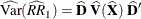whereis the covariance matrix of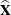,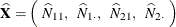andis an array that contains the partial derivatives of the column 1 relative risk with respect to the elements of,